Function Repository Resource:

# GeneralMiniMaxApproximation

Find a mini-max approximation of a function defined parametrically

Contributed by: Wolfram Research
 ResourceFunction["GeneralMiniMaxApproximation"][{fx,fy},{t,{t0,t1},m,n},x] finds the rational polynomial function of x, with numerator order m and denominator order n, that gives a mini-max approximation to the curve with x and y coordinates fx and fy generated as a function of t on the interval t0 to t1. ResourceFunction["GeneralMiniMaxApproximation"][{fx,fy},approx,{t,{t0,t1},m,n},x] finds the mini-max approximation, starting the iterative algorithm with approx.

## Details and Options

ResourceFunction["GeneralMiniMaxApproximation"] by default minimizes the maximum value of the relative error between the approximation and expr.
ResourceFunction["GeneralMiniMaxApproximation"][{fx,fy,g},{t,{t0,t1},m,n},x] computes the error using a factor of g. In this case, the mini-max approximation returned by ResourceFunction["GeneralMiniMaxApproximation"] is the rational function h[x] that minimizes the maximum value of the quantity (fy-h[fx])/g.
ResourceFunction["GeneralMiniMaxApproximation"] returns {abscissa,{approximation,maxerror}}, where abscissa is a list of the abscissas where the relative error is a local maximum, approximation is the rational approximant and maxerror is the global maximum of the relative error.
When an approximation is given as the second argument of ResourceFunction["GeneralMiniMaxApproximation"], it must have the same form as the result returned by ResourceFunction["GeneralMiniMaxApproximation"].
ResourceFunction["GeneralMiniMaxApproximation"] takes the same options as the resource function MiniMaxApproximation.
In the "Derivatives" option, a list of derivatives must give derivatives for both parts of the parametrically-defined function.

## Examples

### Basic Examples (2)

A degree (2,4) mini-max approximation to the inverse of Exp:

 In:=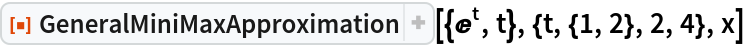Out=Extract the approximation and plot the relative error in the approximation over the interval exe2:

 In:=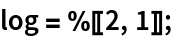In:=Out=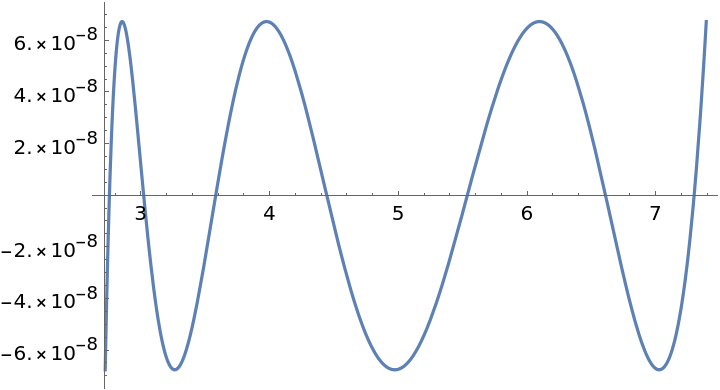Minimize the maximum absolute error:

 In:=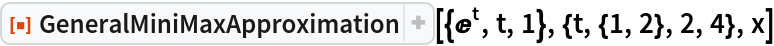Out=The absolute error is equioscillatory:

 In:=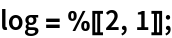In:=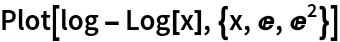Out=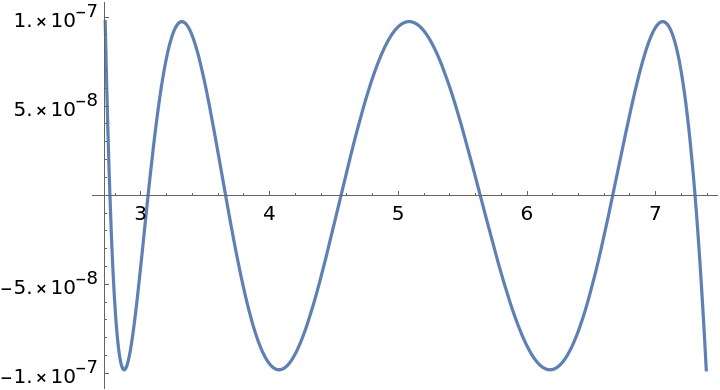### Options (2)

#### Derivatives (2)

A list of derivatives for both parts of parametrically defined function, evaluated at numeric points:

 In:=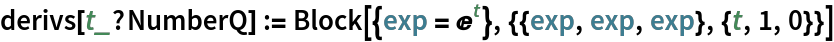The approximation:

 In:=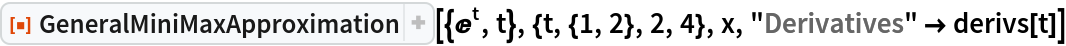Out=### Properties and Relations (1)

For functions with an easily computable inverse, the result given by GeneralMiniMaxApproximation is close to the one returned by the resource function MiniMaxApproximation, up to the abscissas of the extrema:

 In:=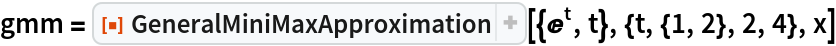Out=In:=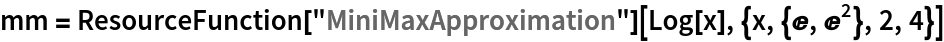Out=In:=Out=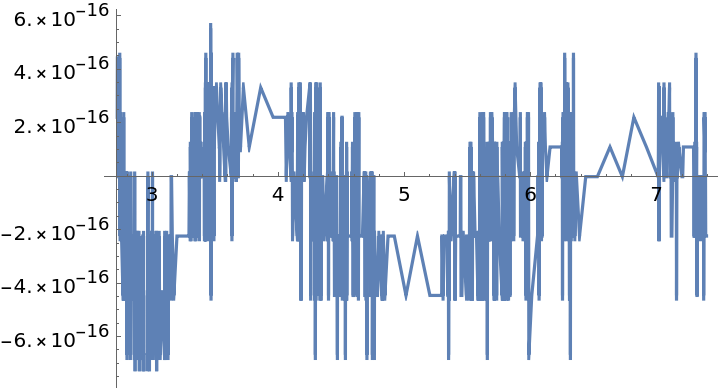## Requirements

Wolfram Language 11.3 (March 2018) or above

## Version History

• 1.0.0 – 29 March 2019